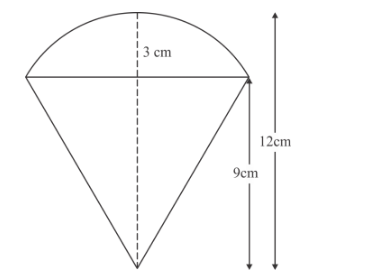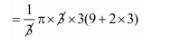# A solid cylinder of diameter 12 cm and height 15 cm`
Question:

A solid cylinder of diameter 12 cm and height 15 cm is melted and recast into toys with the shape of a right circular cone mounted on a hemisphere of radius 3 cm.If the height of the toy is 12 cm, find the number of toys so formed.

Solution:

Diameter of cylinder = 12 cm

Therefore

r = 6 cm

Height = 15 cmTherefore,

Volume of cylinder

$=\pi r^{2} h$

$=36 \times 15 \times \pi \mathrm{cm}^{3}$

Therefore,

Volume of toy = volume of cone + volume of hemisphere

$=\frac{1}{3} \pi r^{2} h+\frac{2}{3} \pi r^{3}$

$=\frac{1}{3} \pi r^{2}(h+2 r)$$=45 \pi \mathrm{cm}^{3}$

Therefore,

No. of toys

$=\frac{\text { volume of cylinder }}{\text { volume of toy }}$

$=\frac{36 \times 15 \times \pi}{45 \times \pi}$

$=12$